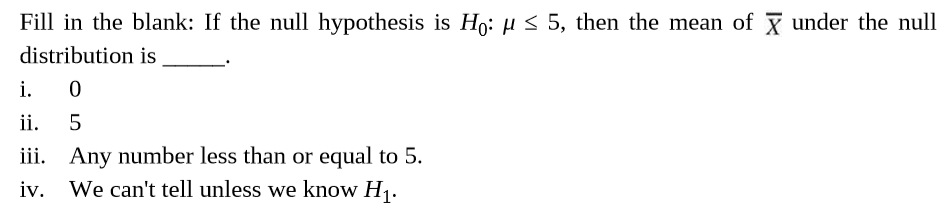# Fill in the blank: If the null hypothesis is Ho: µ < 5, then the mean of x under the nulldistribution isi.ii.iii. Any number less than or equal to 5.iv. We can't tell unless we know H1.

Question
1 viewshelp_outlineImage TranscriptioncloseFill in the blank: If the null hypothesis is Ho: µ < 5, then the mean of x under the null distribution is i. ii. iii. Any number less than or equal to 5. iv. We can't tell unless we know H1. fullscreen
check_circle

Step 1

Points on sampling distribution of the sample mean:

• If the true population distribution of a random variable, say, x, is normal with parameters, mean μ and standard deviation σ, then, whatever be the size (n) of the sample taken from the population, the distribution of the sample mean is also normal, with parameters, mean μ and standard deviation σ/√n.
• Even if the true population distribution of a random variable, say, x, is not normal and has population mean μ, standard deviation σ, then, for a large size (n ≥ 30) of a sample taken from the population, ...

### Want to see the full answer?

See Solution

#### Want to see this answer and more?

Solutions are written by subject experts who are available 24/7. Questions are typically answered within 1 hour.*

See Solution
*Response times may vary by subject and question.
Tagged in